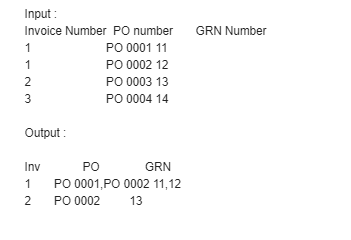# Group two columnsi have attached the screenshot (excel),where i have followed the below steps ,
2.for each row
3.build datatable can anyone help me do this further

Hi @priyankavivek what you are trying to do … if you can explain what you want from the that excel data then we can suggest more clearly.

i need to get the output as shown in screenshot, i need to remove the duplication of invoice number ,and group the 2 columns

Hi @priyankavivek Check this xaml and let me know

_test.xaml (41.1 KB)

Thanks,
Kalyan.

1 Like

Take assign and paste below linq code in it,
DT1 (Type DataTable) =

``````(From p In dta.DefaultView.ToTable(False,"Invoice Number","PO Number","GRN Number").Select Group p By Invoice=Convert.ToString(p("Invoice Number")) Into GroupA=Group Let x=GroupA(0)("Invoice Number").ToString+"|"+String.Join(",",(From p In GroupA Select Convert.ToString(p("PO Number"))).ToArray)+"|"+String.Join(",",(From p In GroupA Select Convert.ToString(p("GRN Number"))).ToArray) Select dta.DefaultView.ToTable(False,"Invoice Number","PO Number","GRN Number").Clone().Rows.Add(x.ToString.split("|"c))).ToArray.CopyToDataTable()
``````

O/P:-here’s the workflow for your understanding.

groupByDisplay.xaml (9.0 KB)i will check and reply on that

okay @priyankavivek